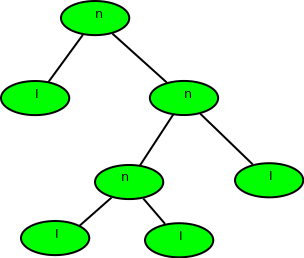Calculate depth of a full Binary tree from Preorder

• Difficulty Level : Medium
• Last Updated : 20 May, 2021

Given preorder of a binary tree, calculate its depth(or height) [starting from depth 0]. The preorder is given as a string with two possible characters.

1. ‘l’ denotes the leaf
2. ‘n’ denotes internal node

The given tree can be seen as a full binary tree where every node has 0 or two children. The two children of a node can ‘n’ or ‘l’ or mix of both.
Examples :

Input  : nlnll
Output : 2
Explanation :Input  : nlnnlll
Output : 3Preorder of the binary tree is given so traverse
Also, we would be given a string of char (formed of ‘n’ and ‘l’), so there is no need to implement tree also.
The recursion function would be:
1) Base Case: return 0; when tree[i] = ‘l’ or i >= strlen(tree)
2) find_depth( tree[i++] ) //left subtree
3) find_depth( tree[i++] ) //right subtree
Where i is the index of the string tree.

C++

 // C++ program to find height of full binary tree// using preorder#include using namespace std; // function to return max of left subtree height// or right subtree heightint findDepthRec(char tree[], int n, int& index){    if (index >= n || tree[index] == 'l')        return 0;     // calc height of left subtree (In preorder    // left subtree is processed before right)    index++;    int left = findDepthRec(tree, n, index);     // calc height of right subtree    index++;    int right = findDepthRec(tree, n, index);     return max(left, right) + 1;} // Wrapper over findDepthRec()int findDepth(char tree[], int n){    int index = 0;    findDepthRec(tree, n, index);} // Driver programint main(){    // Your C++ Code    char tree[] = "nlnnlll";    int n = strlen(tree);     cout << findDepth(tree, n) << endl;     return 0;}

Java

 // Java program to find height// of full binary tree using// preorderimport java .io.*; class GFG{    // function to return max    // of left subtree height    // or right subtree height    static int findDepthRec(String tree,                            int n, int index)    {        if (index >= n ||            tree.charAt(index) == 'l')            return 0;         // calc height of left subtree        // (In preorder left subtree        // is processed before right)        index++;        int left = findDepthRec(tree,                                n, index);         // calc height of        // right subtree        index++;        int right = findDepthRec(tree, n, index);         return Math.max(left, right) + 1;    }     // Wrapper over findDepthRec()    static int findDepth(String tree,                         int n)    {        int index = 0;        return (findDepthRec(tree,                             n, index));    }     // Driver Code    static public void main(String[] args)    {        String tree = "nlnnlll";        int n = tree.length();        System.out.println(findDepth(tree, n));    }} // This code is contributed// by anuj_67.

Python3

 #Python program to find height of full binary tree# using preorder     # function to return max of left subtree height# or right subtree heightdef findDepthRec(tree, n, index) :     if (index >= n or tree[index] == 'l'):        return 0     # calc height of left subtree (In preorder    # left subtree is processed before right)    index += 1    left = findDepthRec(tree, n, index)     # calc height of right subtree    index += 1    right = findDepthRec(tree, n, index)    return (max(left, right) + 1) # Wrapper over findDepthRec()def findDepth(tree, n) :     index =     return findDepthRec(tree, n, index)          # Driver program to test above functionsif __name__ == '__main__':    tree= "nlnnlll"    n = len(tree)     print(findDepth(tree, n)) # This code is contributed by SHUBHAMSINGH10

C#

 // C# program to find height of// full binary tree using preorderusing System; class GFG {     // function to return max of left subtree    // height or right subtree height    static int findDepthRec(char[] tree, int n, int index)    {        if (index >= n || tree[index] == 'l')            return 0;         // calc height of left subtree (In preorder        // left subtree is processed before right)        index++;        int left = findDepthRec(tree, n, index);         // calc height of right subtree        index++;        int right = findDepthRec(tree, n, index);         return Math.Max(left, right) + 1;    }     // Wrapper over findDepthRec()    static int findDepth(char[] tree, int n)    {        int index = 0;        return (findDepthRec(tree, n, index));    }     // Driver program    static public void Main()    {        char[] tree = "nlnnlll".ToCharArray();        int n = tree.Length;        Console.WriteLine(findDepth(tree, n));    }} // This code is contributed by vt_m.

Javascript



Output:

3

Time Complexity: O(N)

Auxiliary Space: O(1)

This article is contributed by Shubham Gupta. If you like GeeksforGeeks and would like to contribute, you can also write an article using write.geeksforgeeks.org or mail your article to contribute@geeksforgeeks.org. See your article appearing on the GeeksforGeeks main page and help other Geeks.